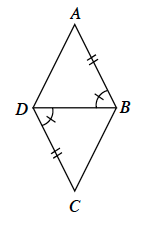### Home > INT1 > Chapter 9 > Lesson 9.3.1 > Problem9-76

9-76.

Determine if the triangles below are congruent. If they are, justify your answer using one of the triangle congruence conditions, and state a sequence of rigid transformations that maps one triangle onto the other.Read Math Note box in Lesson 3.1.4 on rigid transformations.
Read the Math Note box in Lesson 7.1.7 on conditions for triangle congruence.

Remember a congruence statement looks like $Δ\text{____} ≅ Δ\text{_____}$.